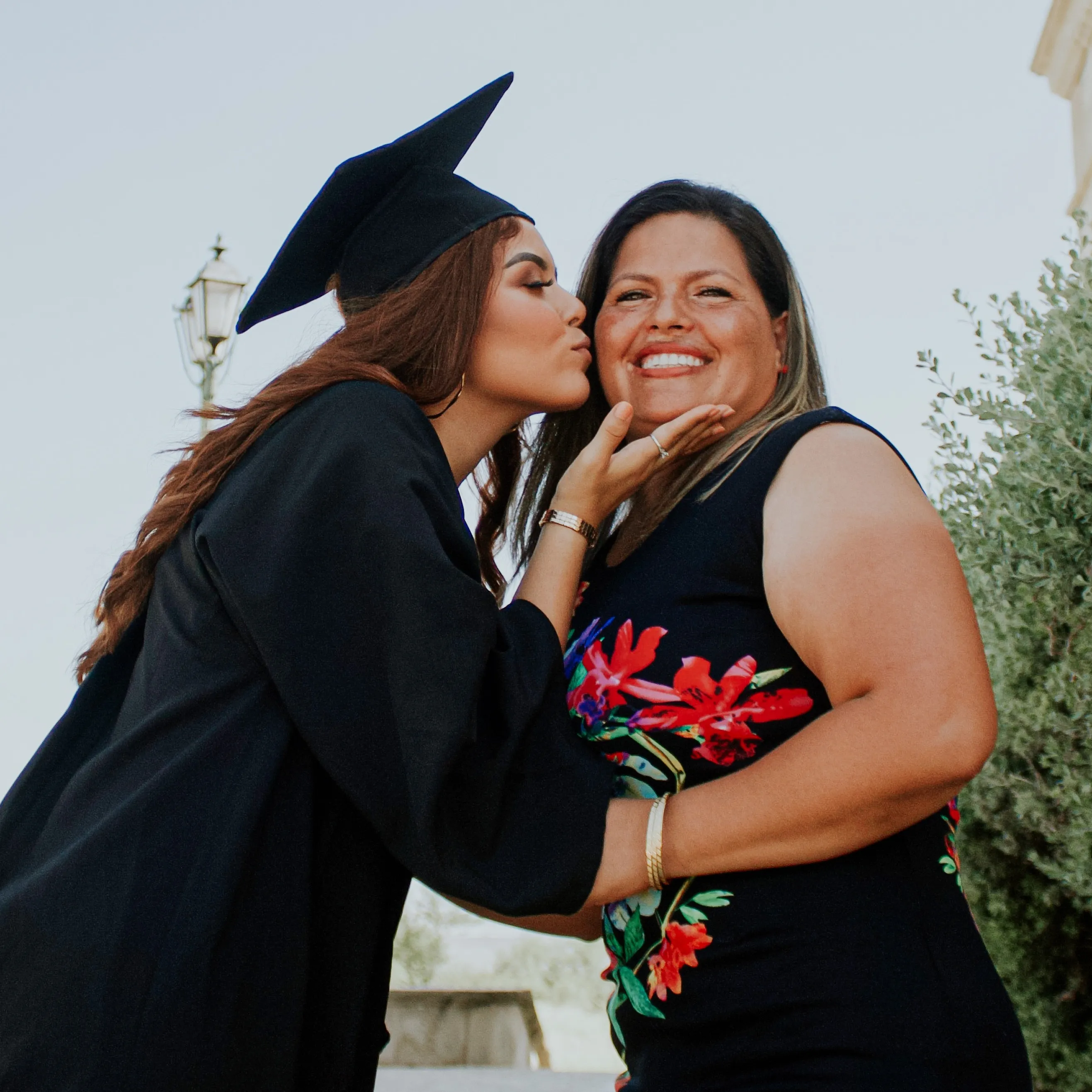I have a bachelor's degree in computer science from the University of Virginia and I will soon earn a doctorate in natural language processing. I have tutored students of extremely diverse backgrounds in grades 3-College. I am very friendly with my students, and this helps them feel comfortable learning with me.

#### My tutoring style:

I have a strong belief that anyone can learn math. Often, students have trouble connecting with their teachers at school. 1-on-1 instruction can definitely help fill in the gaps! I try to meet the students where they’re at. My most important metric is that the students are comfortable and confident in talking and working through problems with me. I put strong emphasis on the basics that will allow the students to have strong fundamentals in order to solve higher-level problems. I like relating math concepts to real-world examples in order to help them ground their learning in a real-world experience. This often helps cement ideas in their brain so that they can build on them productively in the future!

#### Success story:

I was tutoring a high school student in artificial intelligence, and he was having serious trouble coding an optimization algorithm. The algorithm itself is not very complicated, but understanding WHY it works is extremely difficult. We broke it down into a simple game of tic tac toe. By exploring all of the possibilities of the game, we were finally able to recognize why we didn’t have to calculate every board state! Through this dedicated process, the student was able to fully understand the algorithm and finish the course with flying colors.

#### Hobbies and interests:

I played cello for 14 years, formerly did competitive math, and I play chess!

##### K-12 Math
• Elementary Math
• Middle School Math
• Common Core Math
• Singapore Math
• Pre-Algebra
• Algebra I
• Algebra II
• Honors Algebra
• Geometry
• Trigonometry
• Pre-Calculus
• Calculus
• AP Calculus AB
• AP Calculus BC
• Competition Math
##### College Math
• College Algebra
• College Geometry
• College Trigonometry
• College Calculus I
• College Calculus II
• College Calculus III
• Linear Algebra
• Finite Mathematics
• Differential Equations
• Formal Logic
• Combinatorics
• SAT Math
• ACT Math
##### Computer Science
• Computer Science
• AP Computer Science
• Theory of Computer Science
• Java
• Python
• Coding
• Algorithms
• Artificial Intelligence
• Machine Learning

I was tutoring a high school student in artificial intelligence, and he was having serious trouble coding an optimization algorithm. The algorithm itself is not very complicated, but understanding WHY it works is extremely difficult. We broke it down into a simple game of tic tac toe. By exploring all of the possibilities of the game, we were finally able to recognize why we didn’t have to calculate every board state! Through this dedicated process, the student was able to fully understand the algorithm and finish the course with flying colors.

Tutoring subjects:

Elementary Math
Middle School Math
Algebra
Trigonometry
Calculus
AP Calculus
Geometry
College Calculus
Linear Algebra
Differential Equations
Multivariable Calculus
Combinatorics
Formal Logic
ACT Math
SAT Math